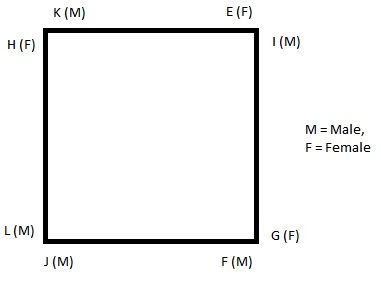(i). Eight persons E, F, G, H, I, J, K and L are seated around a square table -- two on each side.
(ii). There are three lady members and they are not seated next to each other.
(iii). J is between L and F.
(iv). G is between I and F.
(v). H, a lady member, is second to the left of J.
(vi). F, a member is second is seated opposite E, a lady member.
(vii). There is a lady member between F and I.

Which of the following is true about J ?

###### Option:
A. J is a male member.
B. J is a female member,
C. Sex of J cannot be determined.
D. Position of J cannot be determined.
E. None of these
Answer: A . J is a male member.

Justification:

J is between L and F i.e. the order is L J F.
G is between I and F i.e. the order is F G L.
Thus, the sequence becomes L J F G I.
H is second to the left of J i.e. H L J F G L.
Writing the above sequence in form of a square table, we have :E is seated opposite F. Since G is between F and I, so G is a female member.

Clearly, J is a male member.